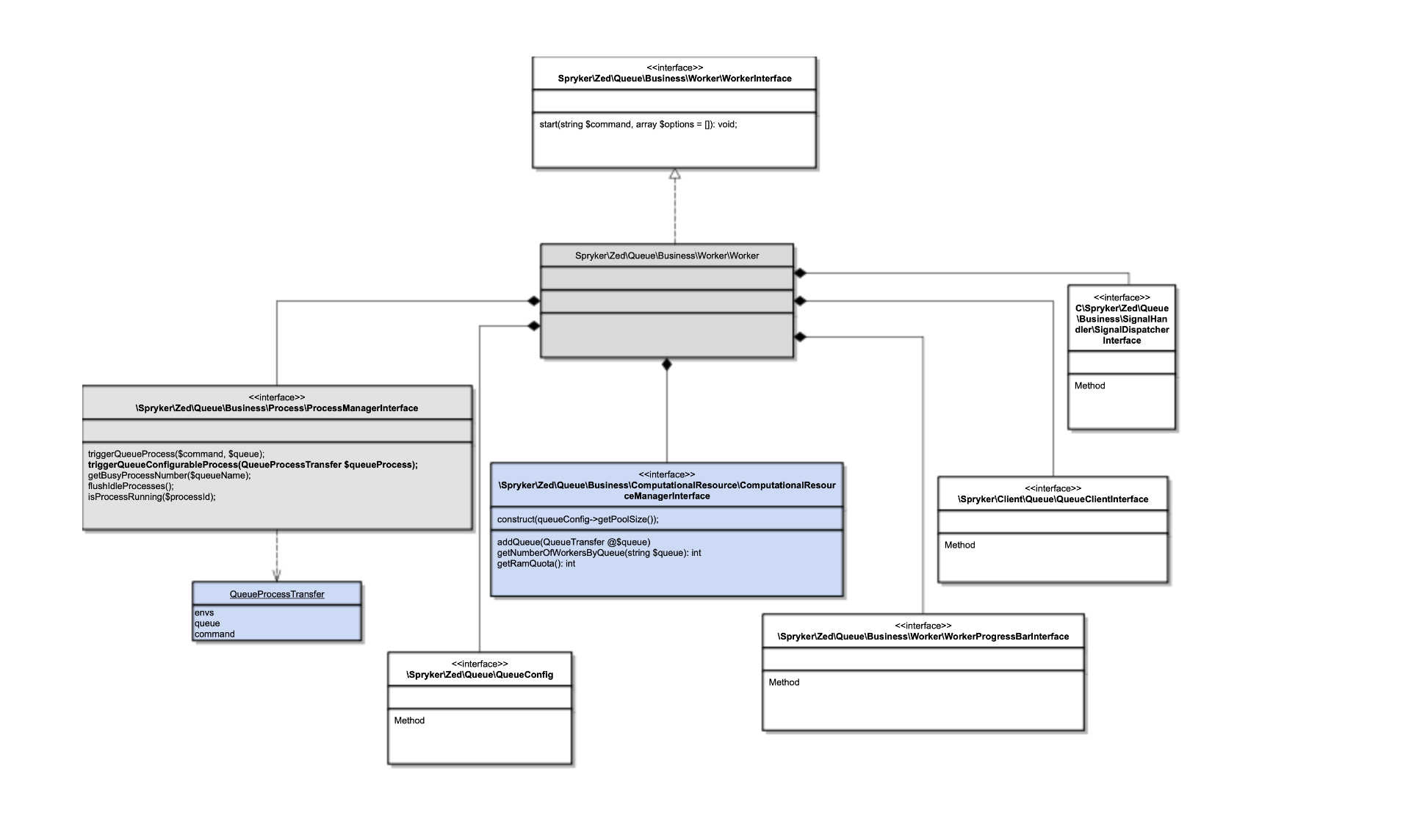Performing compute-intensive operations with significant amount of data without proper resource management inevitably leads to inefficient computation resource utilization and increases processing time.

Scalable application infrastructure improves resource utilization performance and efficiency of the queue processing operation. The computation resource manager component enables efficient distribution of resources among parallel processes.

In the start of processing, each queue contains a different number of messages. To process all messages efficiently, the computational resource manager distributes processes among queues according to the number of messages per queue.

`THREAD_POOL_SIZE` defines the maximum number of parallel processes for all queues. It’s default value is `0`, which means that scalable infrastructure is disabled.

Configuring the right number for `THREAD_POOL_SIZE` affects the resulting scaling behavior and processing efficiency. In general, setting `THREAD_POOL_SIZE` to the number of CPU cores plus one is a good starting point. However, make sure to consider the characteristics of your set of queues and the `max_children` value of your php-fpm server configuration.

Setting `THREAD_POOL_SIZE` to more than `0` disables the `DEFAULT_MAX_QUEUE_WORKER` parameter.

## Query priority

In some cases, a smaller amount of messages may require more workers for processing. To balance such cases, use the `QUEUE_PRIORITY` configurable parameter.

`QUEUE_PRIORITY` defines configurable ratio per queue. If a queue has higher priority in processing, based on this ratio, it allocates more workers in proportion to the number of messages. The default value is `1`.

Configured `QUEUE_PRIORITY` and `THREAD_POOL_SIZE` with consideration of each other may increase efficiency of resource utilization even further. You can fine tune these parameters based on statistics of worker execution. For example, you can collect it using New Relic.

## Workers distribution

For effective worker management, the following calculations are used.

Queue weight is calculated by the formula:

`w = l * p`

Symbols:

• `w`—weight of queue.

• `l`—length of queue.

• `p`—configured queue priority value.

Total weight of all queues is calculated by the formula:

`W = SUM(w)`

Symbols:

• `W`—evaluated total weight of all queues with respect of QUEUE_PRIORITY value.

• `w`—weight of queue.

The number of workers per queue is calculated by the formula:

`n = w * N / W`

Symbols:

• `n`—number of workers for particular queue.

• `N`—thread pool size.

• `w`—weight of queue.

## RAM distribution

To exclude runtime memory limit issues, the memory allocated to each worker is also managed by computational resource manager.

The amount of RAM available for workers is calculated based on `memory_get_usage();` and `ini_get('memory_limit');` and distributed evenly. Formula:

`r = R / N`

Symbols:

• `r`—amount of RAM per worker.

• `R`—total RAM available for worker pool.

• `N`—thread pool size.

## Integrate scalable application infrastructure for publish and sync workers

For instructions, see Integrate scalable application infrastructure for publish and sync workers.

## Implementation details